# Magneton

Also found in: Dictionary, Thesaurus, Medical, Wikipedia.
Related to Magneton: nuclear magneton, Bohr magneton

## Magneton

A unit of magnetic moment used to describe atomic, molecular, or nuclear magnets. More precisely, one unit, the Bohr magneton, is used at the atomic and molecular levels, and another unit, the nuclear magneton, is used at the nuclear level. Still another unit (which might be called the muon magneton, but is usually not named) is used to describe the magnetic moment of the muon.

The Bohr magneton μB is defined and its value given in Eq. (1), where -e and m

(1)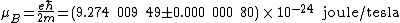are the charge and mass of the electron respectively and &planck; is Planck's constant divided by 2&pgr;. In Dirac's theory the magnetic moment of the electron is exactly -μB, but according to the theory of quantum electrodynamics the electron has a small anomalous magnetic moment. The experimental value of the electron magnetic moment μe is given by Eq. (2), in agreement with the
(2)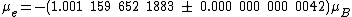prediction of quantum electrodynamics within the errors.

The unit of magnetic moment to describe the muon is obtained from the Bohr magneton by replacing m in Eq. (1) by the muon mass mμ. The experimental value of the muon magnetic moment is given in Eq. (3). The deviation

(3)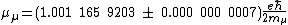of the muon magnetic moment from its Dirac value can also be accounted for by the theory of quantum electrodynamics. See Lepton

The nuclear magneton is obtained from the Bohr magneton by replacing m by the proton mass mp. The value of the nuclear magneton is given in Eq. (4). The

(4)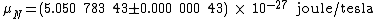nuclear magneton is used not only as the unit for the magnetic moment of the proton but also for the neutron and other hadrons and for atomic nuclei. If the proton and neutron were Dirac particles, the proton's magnetic moment would be one nuclear magneton (except for a small correction arising from quantum electrodynamics) and the neutron's magnetic moment would be zero (because the neutron is uncharged). However, the proton and neutron have large anomalous
(5)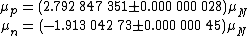magnetic moments, given in Eqs. (5). See Neutron, Nuclear moments, Proton

According to present theory, the proton, neutron, and other hadrons have large anomalous magnetic moments because these particles are not elementary but composite. In the theory of quantum chromodynamics, the principal constituents of a baryon, such as the proton or neutron, are three quarks. See Baryon, Elementary particle, Fundamental constants, Quantum chromodynamics, Quarks

McGraw-Hill Concise Encyclopedia of Physics. © 2002 by The McGraw-Hill Companies, Inc.
The following article is from The Great Soviet Encyclopedia (1979). It might be outdated or ideologically biased.

## Magneton

a unit of measurement of magnetic moment that has been adopted in atomic and nuclear physics.

The magnetic moment of atomic systems is due mainly to the motion of electrons and their spin and is measured in Bohr magnetons: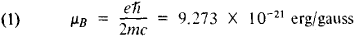Here ħ is Planck’s constant, e and m are the absolute values of the charge and mass of an electron, and c is the speed of light.

In nuclear physics, magnetic moments are measured in nu-clear magnetons, which differ from μB in that the mass of the electron m is replaced by the mass of the proton M: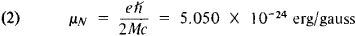The physical meaning of the quantity μB may be understood easily on the basis of a semiclassical examination of the motion of an electron with velocity v in a circular orbit of radius r. This system is analogous to a coil carrying a current whose strength I is equal to the charge divided by the period of rotation: I = ev/2πr. According to classical electrodynamics, in the Gaussian system the magnetic moment of a current-carrying coil that encompasses an area S is μ = IS/c = evr/2c, or μ = eMi/2mc, where M1 — mvr is the orbital angular momentum of the electron. If we take into account the fact that, according to quantum laws, the orbital moment Mi of an electron can assume only discrete values that are multiples of Planck’s constant, Mi = where l = 0, 1, 2,…, then the following expression results: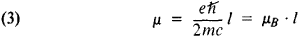Thus, the magnetic moment of an electron in a state with an orbital moment M1 is a multiple of the Bohr magneton. Consequently, in this case μB plays the role of an elementary magnetic moment, a “quantum” of an electron’s magnetic moment.

In addition to the orbital angular momentum M1, which results from rotation, the electron has an intrinsic mechanical moment, the spin, which is s = 1/2 (in units of ħ). The spin magnetic moment is μs = 2μB—that is, it is twice as large as the quantity that would be expected on the basis of formula (3), but since s = 1/2 , μs of an electron is also equal to the Bohr magneton: μs = μB This stems directly from the relativistic quantum theory of the electron, which is based on the Dirac equation.

The nuclear magneton has a similar meaning: it is the magnetic moment created by the motion of a proton (within the nucleus) having an orbital moment l = 1. However, the intrinsic magnetic moments of nuclear particles—the proton and neutron —which, like the electron, have a spin of 1/2, differ significantly from the values that would be expected according to Dirac’s theory. The anomalous magnetic moments of these particles are due to their strong interaction.

D. V. GAL’TSOV

## magneton

[′mag·nə‚tän]
(physics)
A unit of magnetic moment used for atomic, molecular, or nuclear magnets, such as the Bohr magneton, Weiss magneton, or nuclear magneton.
McGraw-Hill Dictionary of Scientific & Technical Terms, 6E, Copyright © 2003 by The McGraw-Hill Companies, Inc.
References in periodicals archive ?
Cine MRI was performed on a 3-Tesla MR scanner (Magneton Symphony Vision, Siemens AG, Erlangen, Germany).
are the Larmor frequencies of the precession of nuclear spin in the ground and excited states with the nuclear g-factors [g.sub.g] and [g.sub.e], respectively, is the nuclear magneton, and [??] = [omega] - [E.sub.0]/h, where [E.sub.0] is the energy of the resonance transition.
All participants were scanned on a 3.0 T Magneton Trio Tim MRI scanner (Siemens Healthcare, Forchheim, Germany).
where S is the electron spin, [g.sub.s] is the spin g-factor and [[mu].sub.B] is the Bohr magneton
Here [alpha] = [e.sup.2]/4[pi] is the electromagnetic fine structure constant, [m.sub.p] is the proton mass, [S.sub.T] is the spin of the target nucleus, and [[??].sub.T] is the magnetic moment of the target nucleus in units of the nuclear magneton e/(2[m.sub.p]) = 0.16 [(GeV/[c.sup.2]).sup.-1].
where [[mu].sub.B] = e[??]/2[m.sub.e] is the Bohr magneton and where [sigma] = ([[sigma].sub.x], [[sigma].sub.y], [[sigma].sub.z]) corresponds to the three Pauli matrices.
The average field-dependence of the FM term can be conveniently reproduced by the Langevin function L(y) = coth(y) - 1/y, assuming unphysically large magnetic moments ("superspins") [[mu].sub.FM] = x[[mu].sub.B], where [[mu].sub.B] is the Bohr magneton and x [much greater than] 1.
where h--Planck constant, v--microwave frequency, [[mu].sub.B]--Bohr magneton, and [B.sub.r]--induction of resonance magnetic field.
g-Factors were calculated from the paramagnetic resonance condition according to the formula : g = hv/[[mu].sub.B][B.sub.r], where h--Planck constant, v--microwave frequency, [[mu].sub.B]-Bohr magneton, and [B.sub.r]--induction of resonance magnetic field.
The magnetic moment for formula unit in Bohr magneton is calculated and the obtained data are displayed in Table 1.Itisseenthat [M.sub.s] decreases as the particle size decreases.

Site: Follow: Share:
Open / Close